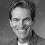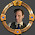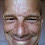## Friday, April 13, 2018

### Fiscal theory of monetary policy

Teaching a PhD class and preparing a few talks led me to a very simple example of an idea, which I'm calling the "fiscal theory of monetary policy." The project is to marry new-Keynesian models, i.e. DSGE models with price stickiness, with the fiscal theory of the price level. The example is simpler than the full analysis with price stickiness in the paper by that title.

It turns out that the FTPL can neatly solve the problems of standard new Keynesian models, and often make very little difference to the actual predictions for time series. This is great news. A new-Keynesian modeler wanting to match some impulse response functions, nervous at the less and less credible underpinnings of new-Keynesian models, can, it appears, just change footnotes about equilibrium selection and get back to work. He or she does not have to throw out a lifetime of work, and start afresh to look at inflation armed with debts and deficits. The interpretation of the model may, however, change a lot.

This is also an extremely conservative (in the non-political sense) approach to curing new-Keynesian model problems. You can keep the entire model, just change some parameter values and solution method, and problems vanish (forward guidance puzzle, frictionless limit puzzle, multiple equilibria at the zero bound, unbelievable off-equilibrium threats etc.) The current NK literature is instead embarked on deep surgery to cure these problems: removing rational expectations, adding constrained or heterogeneous agents, etc. I did not think I would find myself in the strange position trying to save the standard new-Keynesian model, while its developers are eviscerating it! But here we are.

The FTMP model

(From here on in, the post uses Mathjax. It looks great under Chrome, but Safari is iffy. I think I hacked it to work, but if it's mangled, try a different browser. If anyone knows why Safari mangles mathjax and how to fix it let me know.)

Here is the example. The model consists of the usual Fisher equation, $i_{t} = r+E_{t}\pi_{t+1}$ and a Taylor-type interest rate rule $i_{t} = r + \phi \pi_{t}+v_{t}$
$v_{t} =\rho v_{t-1}+\varepsilon_{t}^{i}$
Now we add the government debt valuation equation $\frac{B_{t-1}}{P_{t-1}}\left( E_{t}-E_{t-1}\right) \left( \frac{P_{t-1}% }{P_{t}}\right) =\left( E_{t}-E_{t-1}\right) \sum_{j=0}^{\infty}\frac {1}{R^{j}}s_{t+j}$
Linearizing \begin{equation} \pi_{t+1}-E_{t}\pi_{t+1}=-\left( E_{t}-E_{t+1}\right) \sum_{j=0}^{\infty }\frac{1}{R^{j}}\frac{s_{t+j}}{b_{t}}=-\varepsilon_{t+1}^{s} \label{unexpi} \end{equation} with $$b=B/P$$. Eliminating the interest rate $$i_{t}$$, the equilibrium of this model is now \begin{equation} E_{t}\pi_{t+1} =\phi\pi_{t}+v_{t} \label{epi} \end{equation}
$\pi_{t+1}-E_{t}\pi_{t+1} =-\varepsilon_{t+1}^{s}$
or, most simply, just \begin{equation} \pi_{t+1}=\phi\pi_{t}+v_{t}-\varepsilon_{t+1}^{s}. \label{equil_ftmp} \end{equation}

Here is a plot of the impulse response function:

The top lines plot the response of inflation and interest rates to a unit monetary policy shock $$\varepsilon _{1}^{i}$$ in this model. The line "$$v_{t}$$, FTMP" plots the associated monetary policy disturbance $$v_{t}$$. The combination of two AR(1)s -- the shock persistence $$\rho$$ and the interest rate rule $$\phi$$ -- generates a pretty hump-shaped inflation response. I use $$\rho=0.7$$, $$\phi=0.8$$.

The lower two lines plot the response to a unit fiscal shock $$\varepsilon_{1}^{s}$$. By definition, this disturbance is not persistent. The fiscal tightening produces an instant deflation, i.e. a downward price level jump.

In both cases, the monetary policy $$\phi$$ rule introduces interesting dynamics. Fiscal policy alone sets unexpected inflation $$\pi_{t+1}-E_{t}\pi_{t+1}$$. But what happens after that, $$\left( E_{t+1}-E_{t}\right) \pi_{t+2}$$ and beyond, depends on monetary policy. With a different $$\phi$$, monetary policy could return the price level to its previous value. Monetary policy could turn the event into a one-time price level shock, with no further inflation. Or monetary policy could let the inflation continue for a while, as it does here with $$\phi>0$$.

These responses are not ready to evaluate against data. This model is Fisherian -- higher interest rates via a monetary policy shock produce higher inflation, as they must with a Fisher equation $$i_t = r + E_t \pi_{t+1}$$ and constant $$r$$. The lack of a fiscal change means that $$\pi_{1}$$ cannot jump down. We need to add long term debt and sticky prices, among other ingredients, to produce more realistic response. The important lesson here is that we can produce impulse response functions of this sort, just as we do with standard models of interest rate targets.

The NK model

The simplest form of the standard new-Keynesian model, as set forth for example in Woodford's book, Chapter 2, consists of exactly the same set of equations!

However, new-Keynesian modelers specify that (\ref{unexpi}) determines surpluses $$\left\{ s_{t}\right\}$$ for any unexpected inflation, the "passive" fiscal policy assumption. (Woodford, top of p. 73 for example.) Then, that equation drops out and no longer determines unexpected inflation. To determine unexpected inflation in its place, new-Keynesian modelers specify $$\phi>1$$, and they add a rule against nominal explosions. New-Keynsian authors therefore solve (\ref{epi}) forwards to \begin{equation} \pi_{t}=-E_{t}\sum_{j=0}^{\infty}\frac{v_{t+j}}{\phi_{nk}^{j+1}}=-\sum _{j=0}^{\infty}\frac{\rho_{nk}^{j}}{\phi_{nk}^{j+1}}v_{t}=-\frac{1}{\phi _{nk}-\rho_{nk}}v_{t}. \label{nk_sol} \end{equation}
I use the subscript $$nk$$ here to distinguish parameter values of the two models.

Equilibrium inflation therefore follows the same process as the shock $$v_{t}$$, \begin{equation} \pi_{t+1}=\rho_{nk}\pi_{t}-\frac{1}{ \phi_{nk}-\rho_{nk}}\varepsilon_{t+1}^{i}. \label{equil_nk} \end{equation}
In this simple model, a monetary policy shock $$\varepsilon_{t}^{i}$$ instantly lowers inflation $$\pi_{t}$$. Inflation then recovers back to its steady state with an AR(1) pattern following the slow mean reversion of the disturbance $$v_{t}$$.

The figure above plots the response of this model to a monetary policy shock, using $$\phi_{nk}=1.5$$ and $$\rho_{nk}=0.8$$. The line marked "$$v_{t}$$, NK" plots the resulting monetary policy disturbance, $$v_{t}$$. You can't see the $$\pi_{t}$$ and $$i_{t}$$ lines, because they are exactly the same as the responses of the fiscal theory model to a fiscal $$\varepsilon^{i}$$ shock, using $$\phi=0.8$$. You can also see that fact analytically, comparing (\ref{equil_ftmp}) to (\ref{equil_nk}).

The new-Keynesian model response to a monetary policy shock $$\varepsilon^{i}=(\phi_{nk}-\rho_{nk})$$ in (\ref{equil_nk}) is observationally equivalent to the fiscal theory of monetary policy model response to a (\ref{equil_ftmp}) fiscal shock $$\varepsilon^{s}=1$$, under parameters $$\phi=\rho_{nk}$$, $$\rho=0$$.

Observationally equivalent means observationally equivalent. The response functions are exactly the same. There is no way to tell the two models apart from data on inflation, interest rates, and fiscal surpluses. We do not directly observe underlying shocks or parameter values $$\rho$$ and $$\phi$$. That one model interprets the data via $$\phi \lt 1$$, $$\rho=0$$, and $$\varepsilon^{s}$$ via $$(\ref{equil_ftmp})$$ and the other model interprets the data via $$\phi \gt 1$$,   $$0\lt \rho \lt 1$$ and $$\varepsilon^{i}$$, is not an argument that data can solve.

Repeating what I hope is a familiar point, the relation between equilibrium interest rates and inflation in the new-Keynesian model is $i_t = r + \rho \pi_t .$
A regression of $$i_t$$ on $$\pi_t$$ yields $$\rho$$ not $$\phi$$.

The economics are the same in equilibrium as well. There is an unexpected inflation $$\pi_{t+1}-E_{t}\pi_{t+1}$$ on the day of the shock, because (\ref{unexpi}) holds via "passive" fiscal policy. The new-Keynesian model also has the same fiscal shock, and one could just as well regard the response as really to the fiscal shock. The "monetary policy" shock is a carrot that induces the passive "fiscal policy" horse to disinflate.

The new-Keynesian model is also Fisherian -- interest rates decline here along with the inflation decline. There is no period of high interest rates lowering inflation. Again, we need more ingredients for that.

What the example means

What is the fiscal theory? How does it differ from the standard new-Keynesian theory of monetary policy? How will an applied economist use the fiscal theory? How different are its predictions?

In this example, the answer is that the fiscal theory makes no difference at all to the model's predictions. It amounts to a different understanding about equilibrium formation, and a much different understanding about the course of events and source of shocks underlying a movement such as the bottom two lines of the picture.

More generally, the fiscal theory in a new-Keynesian model only changes the rules for picking unexpected values. Changing the rule for picking unexpected values affects only the impact response, not the shape of subsequent moments of impulse-response functions. For many applied purposes the impact response is not crucial or, as in this example, not identified. In other cases, choosing a different instantaneous response makes a big difference to model predictions. (That's what the "new Keynesian Liquidity Trap" is about.)

The mechanism of equilibrium formation, unimportant for studying data from the equilibrium, is crucially important in thinking about monetary doctrines, and how alternative policy arrangements do and don't work. For example, the fiscal theory and the standard new-Keynesian approach differ on whether an interest rate peg is possible, and more generally whether $$\phi \gt 1$$ or $$\phi \lt 1$$ is desirable. In this FTMP model there is no problem with a peg. $$\phi \gt 1$$ is possible (yes, passive fiscal does not require passive money) but undesirable since inflation will explode.  $$\phi \lt 1$$ is desirable as it leads to stationary inflation. In the standard NK interpretation of the same equations, $$\phi \lt 1$$ leaves unexpected inflation $$\pi_{t+1}-E_t\pi_{t+1}$$ undetermined, so there can be multiple equilibria and sunspots, and $$\phi \gt 1$$, though unobservable, is vital for determinacy. And of course the label and interpretation of the shocks is entirely different. A "monetary policy" shock and a "fiscal policy" shock, though observationally equivalent through the eyes of the two models, have quite a different interpretation.

The standard NK model assumes that the Fed will respond to any inflation it doesn't like by hyperinflating the economy. That threat makes us converge on the one equilibrium that doesn't hyperinflate. The FTMP model frees you from that assumption.

This observational equivalence example strikes me as good news for the fiscal theory project. The basic present value relation invites you to apply the fiscal theory by forming time series of debts and surpluses and looking to them as sources of inflation, rather than by looking at the interest rate decisions of central banks. The fiscal theory has seemed a radical proposal to look at completely different empirical foundations for inflation, with potentially radically different predictions. This example leads to a much more conventional investigation of inflation in terms of interest rate targets, with potentially small differences in testable predictions. Since the only difference between the models is their treatment of innovations, to the extent that expected rather than unexpected inflation dominates its movement in normal times, the "fiscal" part of the fiscal theory will be that much less important.

The fiscal theory becomes a way to maintain the substance of workaday practice with standard new-Keynesian models, while solving in a simple swoop the many holes in its theoretical foundation -- incredible off-equilibrium threats by central bankers, paradoxical policy prescriptions, weird horizon limits and flexible price limits, all of which we will investigate below.

I must admit I'm scratching my head a bit that this is so controversial. Why put so much effort into deep surgery of the model, when just reinterpreting the government debt valuation equation, allows you to solve its problems? Just why is that step so hard? Really all we are doing is adding Pigou effects, wealth effects of government bonds. That step was pretty well accepted some 70 years ago. Why not now?

But the debts and surpluses and the present value formula have not vanished. The fiscal theory offers a unified way of thinking about such "normal times" monetary economics along with the inflations, currency crashes, unconventional policies, and other events in which the fiscal and debt management parts takes center stage, along with deeply different responses to doctrinal and structural or institutional issues.

1.The impulse response looks like ten year treasury yield the from 1976 until a 2016. In 1973 Nixon must have guessed the fiscal theory and tested it such that we could confirm it today, and do it again. It works, after all.

1.Change time a bit. Let depositors over sample and borrowes under, relative to each other. The banker performs term transformation, but has a fixed uncertainty as trades always cause sample rate adaption. We know the sample uncertainty is bound because the uniform sampler model above is optimum in an infinitely wealthy society.

The uniform uncertainty requires the bank to congest the flow, keep matches between depositors and borrowers using large window sizes, enough to measure term length more accurately than the time uncertainty. This is fixed dimensional, the set of relative terms and rate co-adjusting. It is, simply, depositors and borrowers discovering the real 'time to completion' the inside value being priced. The system is orbital, as in semi-repeatable sequences about some random sequence..

2.I let this one through, as it hands down wins the prize for most incoherent comment ever posted to the blog, though in a somewhat humorous way. Is this a computer failing the Turing test?

3.Haha great. Here’s a good line from a bad movie: “At no point in your rambling, incoherent response were you even close to anything that could be considered a rational thought. Everyone in this room is now dumber for having listened to it. I award you no points, and may God have mercy on your soul.”

2.This is cool. Will have to open it up and play with it myself. Thanks for posting this.

1.Careful,

You can go blind doing that :O)

3.Been a while since I passed calculus or constructed models (with Fortran cards).

But let me ask this: Is quantitative easing a way to actually back the currency and reduce the structural deficit as perceived by markets?

The experience with quantitative easing did not seem to lead to inflation in the US, Japan, or Europe.

Of course, Switzerland engaged in a gigantic program of quantitative easing (about \$90,000 per resident) and only manage to prevent further appreciation of its currency.

4.John,

"The new-Keynesian model is also Fisherian -- interest rates decline here along with the inflation decline. There is no period of high interest rates lowering inflation. Again, we need more ingredients for that."

What are those ingredients in the New Keynesian model?

Also, it seems strange for New Keynesian to adopt a Taylor interest raise rule - central bank raises the nominal interest rate to "combat" a rising inflation rate when you just acknowledged that the New Keynesian model is Fisherian - ie, raising the nominal interest rate will increase the inflation rate.

5.The Fischer equation should be:
Interest at t+1 =m Rt + It
R and inflation remain correlated, you can't fix the one without the other. The Fischer is really a Hamiltonian, potential and kinetic trade with each other. In the monetary sense we can see this when it takes three revisions to separate real from inflation in the past. Pricing adjustments is a multi-step process, and over the revision history the marks to market incorporate results from the previous revision. So the monetary shock in 1973 takes about ten steps over the next 50 years. We are just now finalizing the costs of the Reagan military build up, as just one of many examples.

1.In this paper, we propose a procedure based on mixed-frequency models and network analysis to help address both of these policy concerns.

from:
https://www.scribd.com/document/369085349/wp2018-01#fullscreen&from_embed

I am looking at FRB research and they use exactly the method that economic agents use, mainly adjusting the distribution by stepwise adjustment to the network, matched by trelative service intervals at the distribution nodes. From the agents point, the adjustment returns price uncertainty at the give market to return to a well known constant among agents.

Thus, the economy determines how many eggs to a carton, economies of scale.

2.Matt,

"So the monetary shock in 1973 takes about ten steps over the next 50 years."

That suggests a political / fiscal bent to inflation that goes beyond interest rate theory. After all, no one (including the U. S. federal government) borrows for a fixed time frame over more than 30 years.

That being said, the U. S. government does roll over it's existing debt which suggests that the Fisher equation is incomplete because it does not include either the rate of debt growth or the quantity of previously incurred debt.

6.Beautiful graph and discussion of the real interest rate since the 14th century https://voxeu.org/article/suprasecular-stagnation

7.OK, starting to understand this a bit.

Why doesn't the fiscal shock go to negative growth? Because the initial shock was unexpected and resulted in a sudden wealth shift, not a wealth loss.

What happens when the fiscal shock is expected, "happens on a regular basis", say the traders? Then the inflation bounces around zero. Did I get that?

Hence my uncertainty about future prices, finance may be well prepared for the next fiscal shock. Finance and its derivative industry might be preparing us for 1-1.5% deflation for ten years, to get paid back for prior expected inflation losses.

8.Dear respected sir